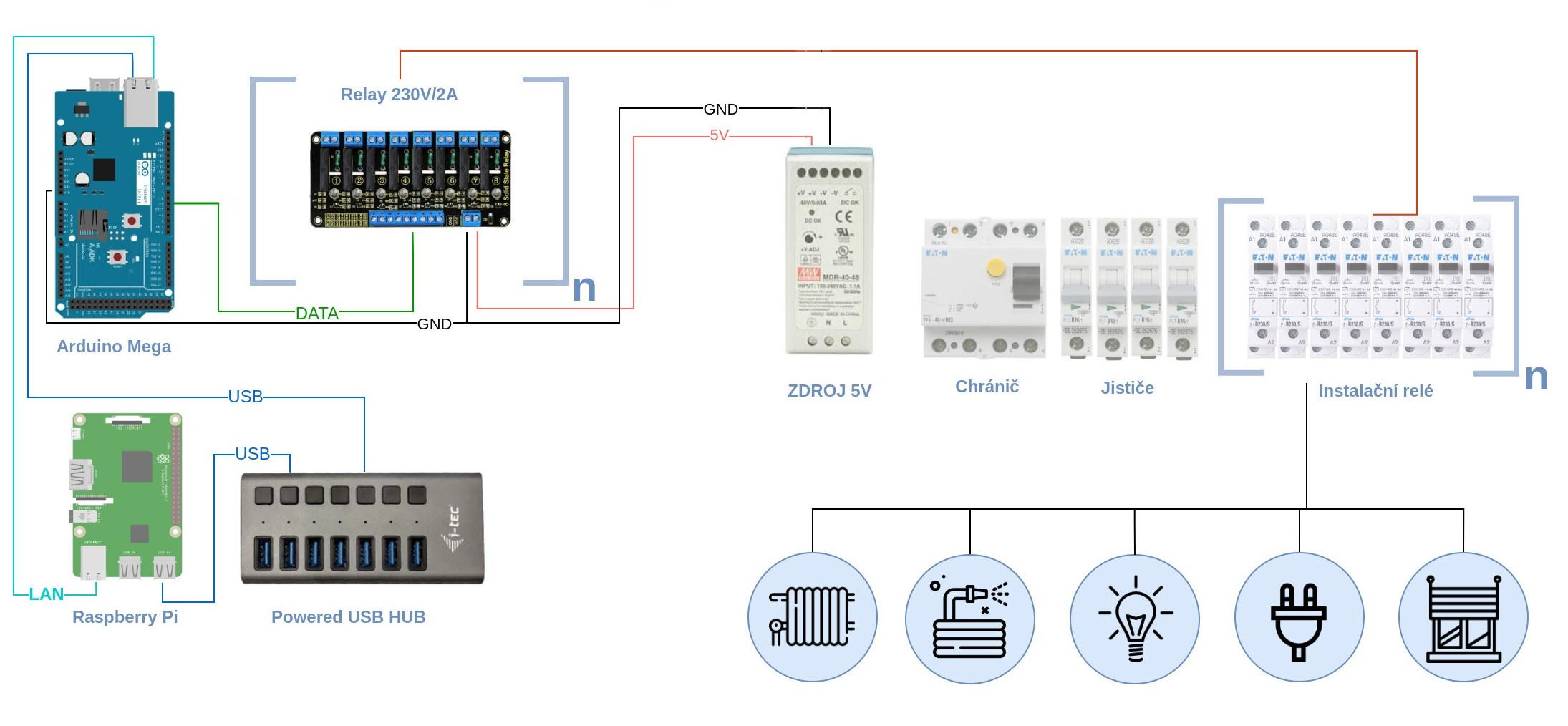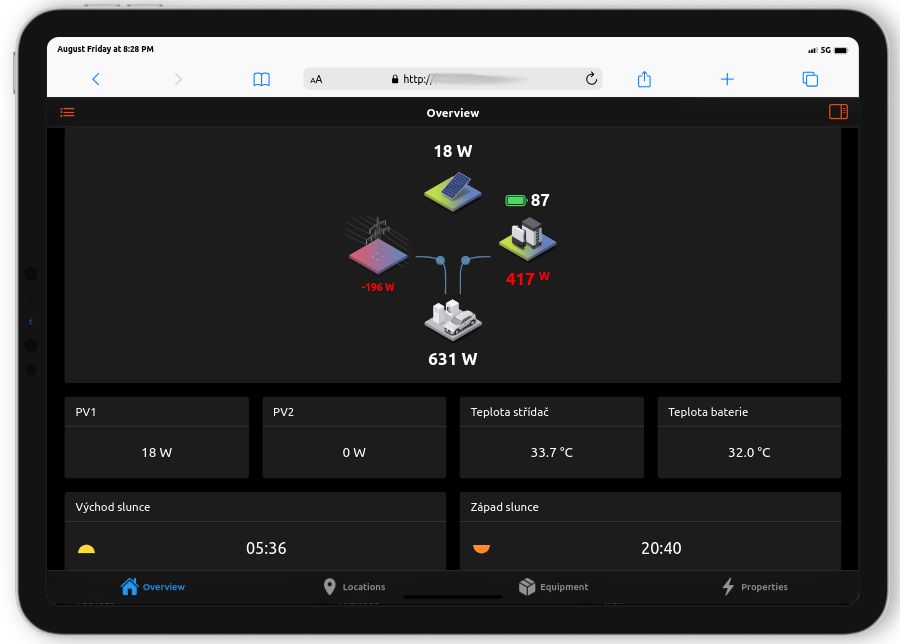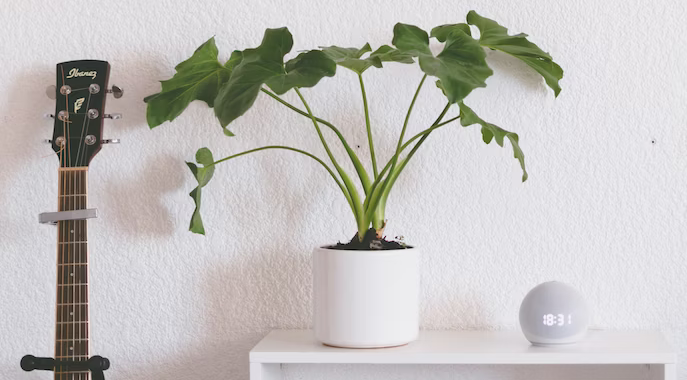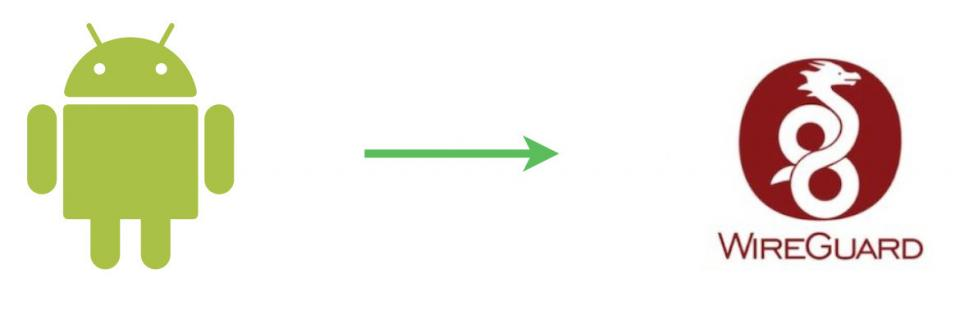## Part 5 - Control power circuits (lights, sockets, heating)

Switching power circuits - sockets, lights, blinds, pumps and other devices under 110-230V is one of the essential functions of an intelligent house. At Pi-Home, we are using Arduino Mega boards with Ethernet Shield, which control Arduino's compatible SSR relays connected with high power relays of the switchboard.

### What we'll need

Part 2 - OpenHAB na Raspberry Pi

Arduino Mega with Ethernet Shield (DIN holder)

Arduino SSR relay 8x2A/230V (DIN holder)

Installation relay (we recommend Hager ERC125S)

DC Power Source 5V 40W (DIN)

### Power and scheme

The Arduino Mega is connected to the RPi via USB and via LAN to the internal network. An 8x2A/230V relay is attached to the Arduino pins. Arduino relay is powered from an external source of DC 5V 40W. Based on the number of controlled outputs, you can place the necessary amount of these relays on the DIN bar and link them to Arduino. These Arduino relays controls the installation relays in the power part of the switchboard. Use multi-phase relays for multi-phase circuits/appliances. High power part of the switchboard should be completed by certified engineer! This is the same as classic home electric appliance.

Tip: "High Trigger" relay is recommended due to safety -> when DC power source failed, it was not start all the inputs as "Low Trigger" modules does.

### Simple scheme for this part### Software

To control the Arduino relay, we provided a simple sketch that subscribe the MQTT communications. In the case of a specific topic and value is subscribed, a relay is switched on and close the circuit. If there are more than one of these relay boards, we recommend having them on a separate Arduino and not combining with sensors or PIRs on one Arduino Mega board.

Example sketch

In this simple sketch SPI.h, Ethernet2.h and PubSubClient.h libraries are used. The Arduino directory must contain a Makefile (filename) with the content below. Makefile contains what libraries we will load and where they are located. You can search libraries online and download. Also, there is a unique Arduino specification in Makefile. We are using a specific name under path /dev/serial/by-id/. You can find there something like usb-Arduinoxxxx. Simply login through SSH to Rasbperry, go to the path /dev/serial/by-id/ and try to plugin/disconnect given Arduino and record what name appears here (ls command) Copy the device name to Makefile below. In the sketch there are declared variables (pins) for relays and the MQTT subscribe logic, some reconnect, etc.

Example of "Makefile"

ARDUINO_DIR   = /usr/share/arduino
AVRDUDE_CONF  = /etc/avrdude.conf
MONITOR_PORT = /dev/serial/by-id/usb-Arduino__www.arduino.cc__0042_8513030353835111C1C0-if00 <--replace green text with your device name under /dev/serial/by-id
USER_LIB_PATH = /usr/share/arduino/libraries/
ARDUINO_LIBS = Ethernet2 SPI pubsubclient
ARDUINO_QUIET = 1
BOARD_TAG = mega
BOARD_SUB = atmega2560
include /usr/share/arduino/Arduino.mk

Example sketch "ArduinoPA1.ino"

/*
Arduino 1 (PA01)

- connects to an MQTT server
- publishes "hello world" to the topic "pihome"
- subscribes to the topic "pihome/xxx"
- controls as many relays as defined
- turns on/off a specific led when it receives a specific "on"/"off" from the "pihome/xxx" topic
- multiple arduino's with same generic sketch can run parallel to each other
- multiple arduino's need each to have a unique ip-addres, unique mac address and unique MQTT client-ID
- tested on arduino-mega with W5500 ethernet shield
*/

//------------------------------------------------------------------------------

#include <SPI.h>
#include <Ethernet2.h>
#include <PubSubClient.h>

// Set relay variables to Arduino digital pins

//PR01
// pin 0 RX
// pin 1 TX
// pin 4 (SS for SD Card)
int L011 = 5;
int L012 = 6;
int L013 = 7;
int L014 = 8;
int L021 = 9;
// pin 10 (SS for Ethernet)
int L022 = 11;
int L031 = 12;
int L041 = 13;

//PR02
int L042 = 2;
int L043 = 3;
int L111 = 14;
int L112 = 15;
int L121 = 16;
int L122 = 17;
int L131 = 18;
int L141 = 19;
// pin 20 SDA
// pin 21 SCL

//PR03
int L151 = 22;
int L152 = 24;
int L153 = 26;
int L154 = 28;
int L155 = 30;
int L156 = 32;
int L161 = 34;
int L201 = 36;
//PR04
int L211 = 23;
int L212 = 25;
int L221 = 27;
int L231 = 29;
int L241 = 31;
int S011 = 33;
int S012 = 35;
int S013 = 37;
//PR05

int S014 = 38;
int S015 = 40;
int S016 = 42;
int S111 = 44;
int S121 = 46;
int S131 = 47;
int S141 = 48;
int S142 = 49;
//PR06
int S151 = 39;
int S152 = 41;
int S153 = 43;
int S154 = 45;

// pin 50 (SS for Ethernet)
// pin 51 (MOSI)
// pin 52 (MISO)
// pin 53 (SCK)

//PR07
//A0 (D54)
// int Rez = 54;
// int Rez = 55;
// int Rez = 56;
// int Rez = 57;
// int Rez = 58;
// int Rez = 59;
// pin 60 inaccesibble
// int Rez = 61;

int S155 = 63;
int S161 = 64;
int S201 = 65;
int S211 = 66;
int S221 = 67;
int S231 = 68;
int S241 = 69;
//A15 (D69)

// Set variables to act as virtual switches
// Set variable values initially to LOW (and not HIGH)
// HIGH or LOW as defautl is based on Relay Board

//PR01
int ValL011 = LOW;
int ValL012 = LOW;
int ValL013 = LOW;
int ValL014 = LOW;
int ValL021 = LOW;
int ValL022 = LOW;
int ValL031 = LOW;
int ValL041 = LOW;
//PR02
int ValL042 = LOW;
int ValL043 = LOW;
int ValL111 = LOW;
int ValL112 = LOW;
int ValL121 = LOW;
int ValL122 = LOW;
int ValL131 = LOW;
int ValL141 = LOW;
//PR03
int ValL151 = LOW;
int ValL152 = LOW;
int ValL153 = LOW;
int ValL154 = LOW;
int ValL155 = LOW;
int ValL156 = LOW;
int ValL161 = LOW;
int ValL201 = LOW;
//PR04
int ValL211 = LOW;
int ValL212 = LOW;
int ValL221 = LOW;
int ValL231 = LOW;
int ValL241 = LOW;
//int REZ=

//PR05
int ValS011 = LOW;
int ValS012 = LOW;
int ValS013 = LOW;
int ValS014 = LOW;
int ValS015 = LOW;
int ValS016 = LOW;
int ValS111 = LOW;
//PR06
int ValS121 = LOW;
int ValS131 = LOW;
int ValS141 = LOW;
int ValS142 = LOW;
int ValS151 = LOW;
int ValS152 = LOW;
int ValS153 = LOW;
int ValS154 = LOW;

//PR07
int ValS155 = LOW;
int ValS161 = LOW;
int ValS201 = LOW;
int ValS211 = LOW;
int ValS221 = LOW;
int ValS231 = LOW;
int ValS241 = LOW;

//---------------------------------------------------------------------------

// Arduino MAC address must be unique for every node in same network
// To make a new unique address change last letter
// Arduino 1
byte mac[]    = { 0xCC, 0xFA, 0x0C, 0xCA, 0x19, 0x01 };

// Unique static IP address of this Arduino 1

byte server[] = { 10, 0, 0, 24 };

// Handle and convert incoming MQTT messages ----------------------------------------

void callback(char* topic, byte* payload, unsigned int length) {
// handle message arrived
String content="";
char character;
for (int num=0;num<length;num++) {
content.concat(character);
}

//Relay Power
// Set specific virtual switches on basis of specific incoming messages ----------------------------

if (content == "L011_on")  { ValL011 =  HIGH; }
if (content == "L011_off") { ValL011 =  LOW; }
if (content == "L012_on")  { ValL012 =  HIGH; }
if (content == "L012_off") { ValL012 =  LOW; }
if (content == "L013_on")  { ValL013 =  HIGH; }
if (content == "L013_off") { ValL013 =  LOW; }
if (content == "L014_on")  { ValL014 =  HIGH; }
if (content == "L014_off") { ValL014 =  LOW; }
if (content == "L021_on")  { ValL021 =  HIGH; }
if (content == "L021_off") { ValL021 =  LOW; }
if (content == "L022_on")  { ValL022 =  HIGH; }
if (content == "L022_off") { ValL022 =  LOW; }
if (content == "L031_on")  { ValL031 =  HIGH; }
if (content == "L031_off") { ValL031 =  LOW; }
if (content == "L041_on")  { ValL041 =  HIGH; }
if (content == "L041_off") { ValL041 =  LOW; }

if (content == "L042_on")  { ValL042 =  HIGH; }
if (content == "L042_off") { ValL042 =  LOW; }
if (content == "L043_on")  { ValL043 =  HIGH; }
if (content == "L043_off") { ValL043 =  LOW; }
if (content == "L111_on")  { ValL111 =  HIGH; }
if (content == "L111_off") { ValL111 =  LOW; }
if (content == "L112_on")  { ValL112 =  HIGH; }
if (content == "L112_off") { ValL112 =  LOW; }
if (content == "L121_on")  { ValL121 =  HIGH; }
if (content == "L121_off") { ValL121 =  LOW; }
if (content == "L122_on")  { ValL122 =  HIGH; }
if (content == "L122_off") { ValL122 =  LOW; }
if (content == "L131_on")  { ValL131 =  HIGH; }
if (content == "L131_off") { ValL131 =  LOW; }
if (content == "L141_on")  { ValL141 =  HIGH; }
if (content == "L141_off") { ValL141 =  LOW; }

if (content == "L151_on")  { ValL151 =  HIGH; }
if (content == "L151_off") { ValL151 =  LOW; }
if (content == "L152_on")  { ValL152 =  HIGH; }
if (content == "L152_off") { ValL152 =  LOW; }
if (content == "L153_on")  { ValL153 =  HIGH; }
if (content == "L153_off") { ValL153 =  LOW; }
if (content == "L154_on")  { ValL154 =  HIGH; }
if (content == "L154_off") { ValL154 =  LOW; }
if (content == "L155_on")  { ValL155 =  HIGH; }
if (content == "L155_off") { ValL155 =  LOW; }
if (content == "L156_on")  { ValL156 =  HIGH; }
if (content == "L156_off") { ValL156 =  LOW; }
if (content == "L161_on")  { ValL161 =  HIGH; }
if (content == "L161_off") { ValL161 =  LOW; }
if (content == "L201_on")  { ValL201 =  HIGH; }
if (content == "L201_off") { ValL201 =  LOW; }

if (content == "L211_on")  { ValL211 =  HIGH; }
if (content == "L211_off") { ValL211 =  LOW; }
if (content == "L212_on")  { ValL212 =  HIGH; }
if (content == "L212_off") { ValL212 =  LOW; }
if (content == "L221_on")  { ValL221 =  HIGH; }
if (content == "L221_off") { ValL221 =  LOW; }
if (content == "L231_on")  { ValL231 =  HIGH; }
if (content == "L231_off") { ValL231 =  LOW; }
if (content == "L241_on")  { ValL241 =  HIGH; }
if (content == "L241_off") { ValL241 =  LOW; }

if (content == "S011_on")  { ValS011 =  HIGH; }
if (content == "S011_off") { ValS011 =  LOW; }
if (content == "S012_on")  { ValS012 =  HIGH; }
if (content == "S012_off") { ValS012 =  LOW; }
if (content == "S013_on")  { ValS013 =  HIGH; }
if (content == "S013_off") { ValS013 =  LOW; }
if (content == "S014_on")  { ValS014 =  HIGH; }
if (content == "S014_off") { ValS014 =  LOW; }
if (content == "S015_on")  { ValS015 =  HIGH; }
if (content == "S015_off")  { ValS015 =  LOW; }
if (content == "S016_on")  { ValS016 =  HIGH; }
if (content == "S016_off") { ValS016 =  LOW; }
if (content == "S111_on")  { ValS111 =  HIGH; }
if (content == "S111_off") { ValS111 =  LOW; }

if (content == "S121_on")  { ValS121 =  HIGH; }
if (content == "S121_off") { ValS121 =  LOW; }
if (content == "S131_on")  { ValS131 =  HIGH; }
if (content == "S131_off") { ValS131 =  LOW; }
if (content == "S141_on")  { ValS141 =  HIGH; }
if (content == "S141_off") { ValS141 =  LOW; }
if (content == "S142_on")  { ValS142 =  HIGH; }
if (content == "S142_off") { ValS142 =  LOW; }
if (content == "S151_on")  { ValS151 =  HIGH; }
if (content == "S151_off") { ValS151 =  LOW; }
if (content == "S152_on")  { ValS152 =  HIGH; }
if (content == "S152_off") { ValS152 =  LOW; }
if (content == "S153_on")  { ValS153 =  HIGH; }
if (content == "S153_off") { ValS153 =  LOW; }
if (content == "S154_on")  { ValS154 =  HIGH; }
if (content == "S154_off") { ValS154 =  LOW; }

if (content == "S155_on")  { ValS155 =  HIGH; }
if (content == "S155_off") { ValS155 =  LOW; }
if (content == "S161_on")  { ValS161 =  HIGH; }
if (content == "S161_off") { ValS161 =  LOW; }
if (content == "S201_on")  { ValS201 =  HIGH; }
if (content == "S201_off") { ValS201 =  LOW; }
if (content == "S211_on")  { ValS211 =  HIGH; }
if (content == "S211_off") { ValS211 =  LOW; }
if (content == "S221_on")  { ValS221 =  HIGH; }
if (content == "S221_off") { ValS221 =  LOW; }
if (content == "S231_on")  { ValS231 =  HIGH; }
if (content == "S231_off") { ValS231 =  LOW; }
if (content == "S241_on")  { ValS241 =  HIGH; }
if (content == "S241_off") { ValS241 =  LOW; }

// Set digital pin states according to virtual switch settings

digitalWrite(L011, ValL011);
digitalWrite(L012, ValL012);
digitalWrite(L013, ValL013);
digitalWrite(L014, ValL014);
digitalWrite(L021, ValL021);
digitalWrite(L022, ValL022);
digitalWrite(L031, ValL031);
digitalWrite(L041, ValL041);

digitalWrite(L042, ValL042);
digitalWrite(L043, ValL043);
digitalWrite(L111, ValL111);
digitalWrite(L112, ValL112);
digitalWrite(L121, ValL121);
digitalWrite(L122, ValL122);
digitalWrite(L131, ValL131);
digitalWrite(L141, ValL141);

digitalWrite(L151, ValL151);
digitalWrite(L152, ValL152);
digitalWrite(L153, ValL153);
digitalWrite(L154, ValL154);
digitalWrite(L155, ValL155);
digitalWrite(L156, ValL156);
digitalWrite(L161, ValL161);
digitalWrite(L201, ValL201);

digitalWrite(L211, ValL211);
digitalWrite(L212, ValL212);
digitalWrite(L221, ValL221);
digitalWrite(L231, ValL231);
digitalWrite(L241, ValL241);

digitalWrite(S011, ValS011);
digitalWrite(S012, ValS012);
digitalWrite(S013, ValS013);
digitalWrite(S014, ValS014);
digitalWrite(S015, ValS015);
digitalWrite(S016, ValS016);
digitalWrite(S111, ValS111);

digitalWrite(S121, ValS121);
digitalWrite(S131, ValS131);
digitalWrite(S141, ValS141);
digitalWrite(S142, ValS142);
digitalWrite(S151, ValS151);
digitalWrite(S152, ValS152);
digitalWrite(S153, ValS153);
digitalWrite(S154, ValS154);

digitalWrite(S155, ValS155);
digitalWrite(S161, ValS161);
digitalWrite(S201, ValS201);
digitalWrite(S211, ValS211);
digitalWrite(S221, ValS221);
digitalWrite(S231, ValS231);
digitalWrite(S241, ValS241);

}

// Initiate instances -----------------------------------

EthernetClient arduino1;
PubSubClient client(server, 1883, callback, arduino1);

void setup()
{

digitalWrite(L011, LOW);
digitalWrite(L012, LOW);

pinMode(L011, OUTPUT);
pinMode(L012, OUTPUT);

// Setup ethernet connection to MQTT broker
Ethernet.begin(mac, ip);
if (client.connect("arduino1", "openhabian", "openhabian")) {
client.publish("pihome","Hello world - here Arduino1 with IP 10.0.0.171");
client.subscribe("pihome/lights");
client.subscribe("pihome/sockets");

}
}

//-----------------------------------------------
long lastReconnectAttempt = 0;

boolean reconnect() {
if (client.connect("arduino1", "openhabian", "openhabian")) {
// Once connected, publish an announcement...
client.publish("pihome","Arduino 1 - reconnected");
client.subscribe("pihome/lights");
client.subscribe("pihome/sockets");
}
return client.connected();
}
//----------------------------------------------

void loop()
{

if (!client.connected()) {
long now = millis();
if (now - lastReconnectAttempt > 5000) {
lastReconnectAttempt = now;
// Attempt to reconnect
if (reconnect()) {
lastReconnectAttempt = 0;
}
}
} else {
// Client connected

client.loop();
}

}

// End of sketch ---------------------------------

### OpenHAB 3

In OpenHAB, you should already have Items represent lights and sockets from previous part of tutorial. Try to turn on/off some of them and your realys should react on your inputs. We have experience with hundreads of controlled circuits for a 10 years without any issues.

Tip: One Arduino Mega board with Ethernet shield you can connect up to 6 pieces of 8x SSR relay board which is up to 48 lights/sockets/pumps etc.

### Nejlepší články z bloguSmart Home vs GoodWeRating:
50%

In the post, we will demonstrate step by step how to communicate directly with the GoodWe inverter in a smart home setup and obtain real-time information (unlike the SEMS portal). This information is essential if we want to react to current parameters in a smart home, such as activating additional cooling or controlling a socket with a various load.Voice control of the houseRating:
0%

In this article, we will connect the Amazon Echo Dot voice assistant with open source home automation. We won't be using OpenHAB Cloud, so everything runs locally. In this case, a few additional settings are necessary, but the result is worth it!Basics - Winbox, DHCPRating:
65.9%

In this series, we will look at the step-by-step setup of MikroTik devices for home users or a small business (up to 25 people). In the first article, we will focus on the initial setup - we will download Winbox and set up DHCP for the primary network and guest network. Similarly, we will also adjust the WiFi settings.Alarm from existing PIR sensors in a smart home.Rating:
0%

In a your smart home, PIR sensors may not only be used to switch lights on and off based on motion, it is possible to utilize these sensors to detect the presence of motion in a particular room. This information can be used to create a relatively reliable uncertified home security system. In this guide, you will find the logic for how this can work in the OpenHAB software in our model smart home.NFC tags in smart homeRating:
80%

NFC (Near Field Communication) tags are small plastic or paper stickers that can be used to automate various functions in the smart home. In this article, we will show you examples of use and a guide on how to write an action on an NFC tag using a mobile phone.WireGuard on iOS devicesRating:
85%

In this article, you will find a detailed guide on how to connect to WireGuard VPN from iOS.WireGuard on Android devicesRating:
0%

In this article, you will find a detailed guide on how to connect to WireGuard VPN from Android.WireGuard on MikroTikRating:
90%

This article describes the self setup of the WireGuard VPN protocol on MikroTik devices with RouterOS version 7 and higher. This phenomenal VPN is very fast, secure, and easily configurable in a home environment. It can be said that it is currently the best VPN for home use available.Nilan VP18 vs OpenHABRating:
67.2%

We provide tutorial for the connection of the active Nilan recuperation unit, in our case the VP18 K WT type with OpenHAB 3. By communicating via Modbus it is possible to read the whole range of values, but mainly also to control the unit through OpenHAB. This allows us to remotely or programmatically change the performance and ventilation settings depending on the mode (night, cooking, etc.)Energy meter in smart homeRating:
70.3%

We are providing a simple step-by-step tutorial how to install home energy meter. We did not find any MQTT device, so we buy Aeotec Home Energy Meter as we are already have a Z-Wave controlller in our smart home.